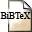# Optimum positioning of base stations for cellular radio networks

## Abstract

Finding optimum base station locations for a cellular radio network is considered as a mathematical optimization problem. Dependent on the channel assignment policy, the minimization of interferences or the number of blocked channels, respectively, may be more favourable. In this paper, a variety of according analytical optimization problems is introduced. Each is formalized as an integer linear program, and in most cases optimum solutions can be given. Whenever by the complexity of the problem an exact solution is out of reach, simulated annealing is used as an approximate optimization technique. The performance of the different approaches is compared by extensive numerical tests.

## BibTEX Reference Entry```@article{MaNi00,
author = {Rudolf Mathar and Thomas Niessen},
title = "Optimum positioning of base stations for cellular radio networks",
pages = "421-428",
journal = "Wireless Networks",
volume = "6",
number = "4",
year = 2000,
hsb = RWTH-CONV-223166,
}
```Download bibtex-file